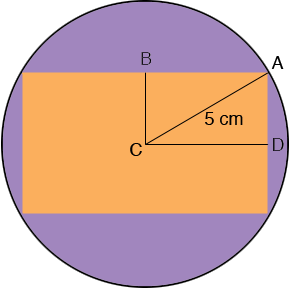SEARCH HOMEMath Central Quandaries & QueriesQuestion from sridhar, a student: A rectangle with perimeter 28 cm inscribed in a circle of radius 5 cm find the area ?Sridhar,If the perimeter of the rectangle is 28 cm then the length of BA plus the length of AD is 1/4 of 28 or 7 cm. Thus if one of them, say BA has length x cm then the other, AD had length 7 - x cm. Write down what Pythagoras' theorem tell you about the triangle ABC, and solve for x.

PennyMath Central is supported by the University of Regina and The Pacific Institute for the Mathematical Sciences.